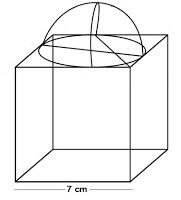Newbie

# A cubical block of side 7 cm is surmounted by a hemisphere. What is the greatest diameter the hemisphere can have? Find the surface area of the solid. Q.4

• 1

Find the solution of surface areas and volumes chapter of class 10th ncert how is solve the exercise 13.1 question number 4 give the best solution of this question ,please help me to find out the correct way to put the formula in that question .A cubical block of side 7 cm is surmounted by a hemisphere. What is the greatest diameter the hemisphere can have? Find the surface area of the solid.

Share

1. It is given that each side of cube is 7 cm. So, the radius will be 7/2 cm.We know,

The total surface area of solid (TSA) = surface area of cubical block + CSA of hemisphere – Area of base of hemisphere

∴ TSA of solid = 6×(side)2+2πr2-πr2

= 6×(side)2+πr2

= 6×(7)2+(22/7)×(7/2)×(7/2)

= (6×49)+(77/2)

= 294+38.5 = 332.5 cm2

So, the surface area of the solid is 332.5 cm2

• 0